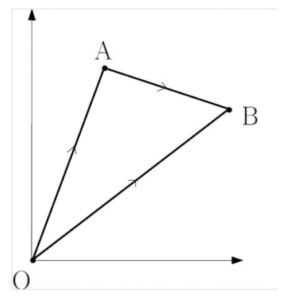# Vectors in Context

## Position Vectors

A position vector is any vector that is placed extending from the origin. Position vectors are often denoted by $\overrightarrow{OA}$, for example, to identify the vector that points from the origin to a point $A$.Let $\overrightarrow{OA}$ and $\overrightarrow{OB}$ be the position vectors that point from the origin to the points $A$ and $B$ respectively. We can then find the vector that points from $A$ to $B$:

$\overrightarrow{AB}=\overrightarrow{OB}-\overrightarrow{OA}$

It is easy to visualise going from $A$ to $B$ by thinking of it as going backwards along $\overrightarrow{OA}$, then forwards along $\overrightarrow{OB}$.

There are a variety of problems involving position vectors. Examples 1 and 2 illustrate just two ways in which position vectors can be put into context.

## Vectors and Trigonometry

By now you will be very familiar with Pythagoras and SOHCAHTOA. This allows you to find angles and missing lengths in right-angled triangles. You may also have recently learned about non-right angled triangles. That is to say, you may have learned about the cosine rule, the sine rule and perhaps even how to find the area of a non-right angled triangle.

It is entirely possible that trigonometry may be used in the context of vectors. Examples 3 and 4 illustrate just two ways in which vectors can be using in a trigonometric setting.

## Examples

The points $A$ and $B$ have position vectors $3{\bf i}-4{\bf j}$ and $2{\bf j}-7{\bf I}$ respectively. The point $P$ splits the line vector $\overrightarrow{AB}$ in the ratio 2:3. Find the position vector $\overrightarrow{OP}$.

A postman picks up his letters from the depot (point $O$). He then heads west delivering letters for 2 km (to point $A$) before heading south down a road for 5 km (to point $B$). Calculate:

1. his position vector at the end of his route relative to the depot, i.e. the vector $\overrightarrow{OB}$,
2. the distance (as the crow flies) between the postman at the end of the route and the depot,
3. and the bearing of the postman at the end of his route from the depot.

A ship leaves a port and heads to its destination for 65 km on a bearing of $071^\circ$ before receiving a distress signal. The distress signal is sent from a vessel with a position that is 54km away from and at a bearing of $122^\circ$ relative to the port. Calculate the distance between the vessel in distress and the ship.